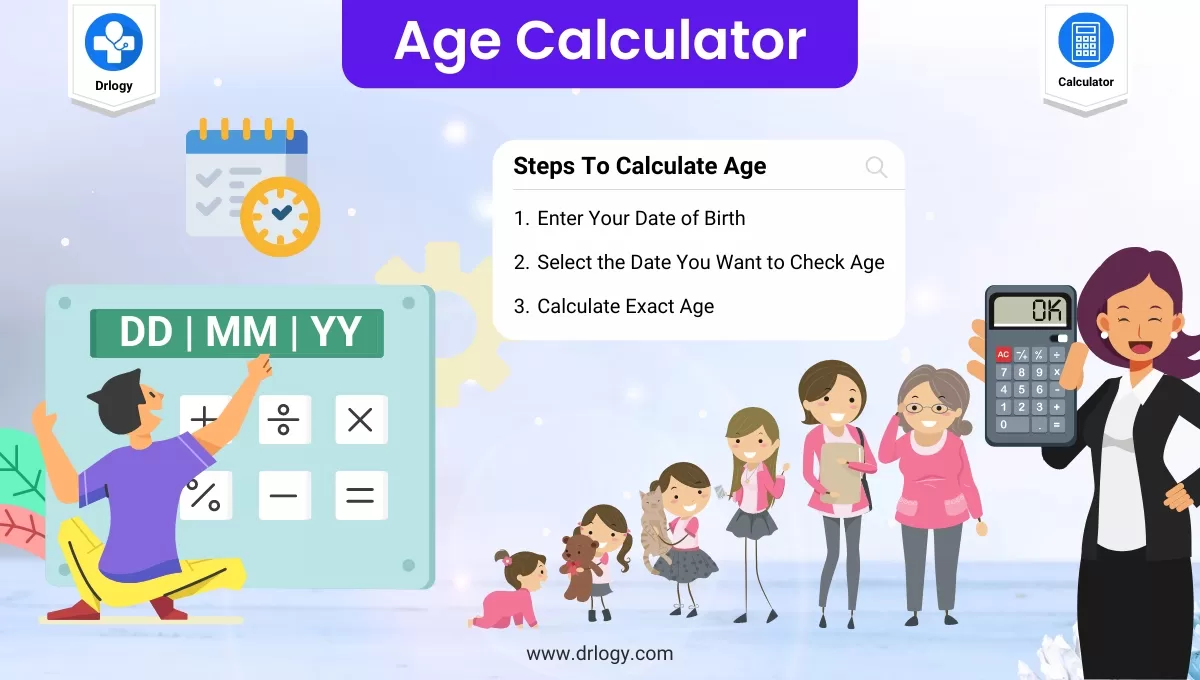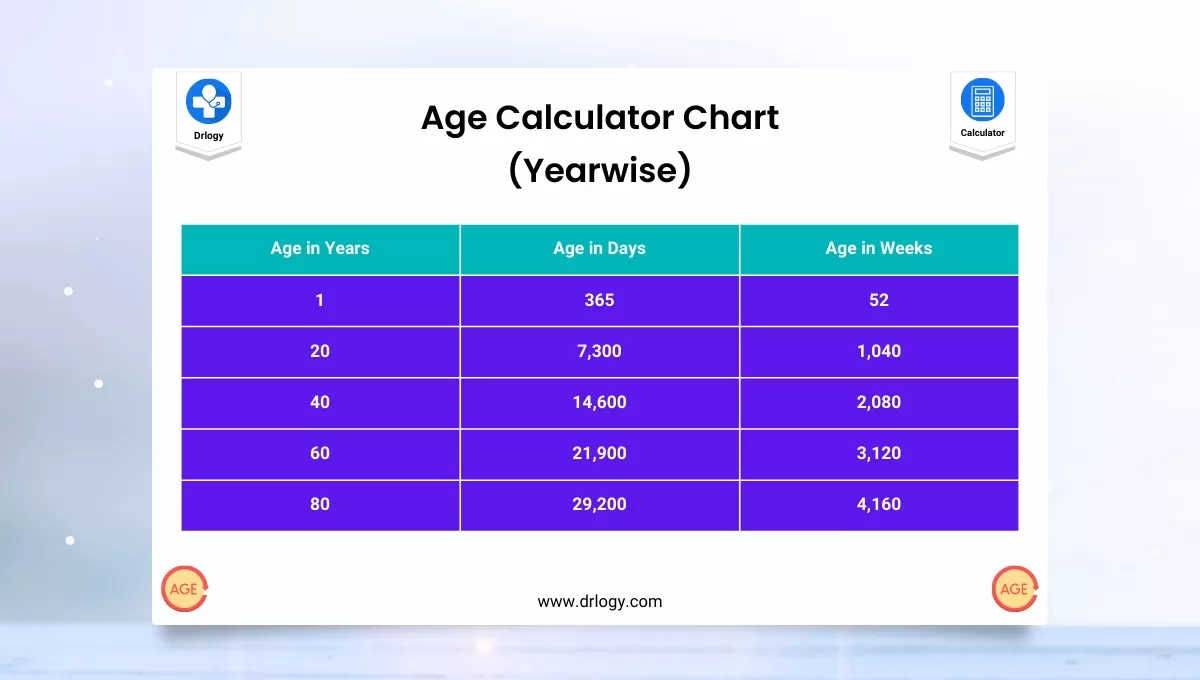• Health
• Pet
• Unit Conversion
• Medical# Age Calculator Online - Calculate Age From Date Of Birth

## Age Calculator

###### Result
• Age
• 0 years 0 months 0 days
• 0 months 0 days
• 0 weeks 0 days
• 0 days
• 0 hours
• 0 minutes
• 0 seconds

## What is Age Calculator?

• The Age Calculator will determine the age or interval between two dates.
• The calculated age will be displayed in years, months, weeks, days, hours, minutes, and seconds.

## Age Calculator Use

• Calculate the age of a person, place, or thing.
• The age calculator calculates age given a date of birth in years, months, and days.
• You can also use this calculator to find the length of time between two dates.
• The age calculator finds the age time span in years months and days, months and days, and in total days only.

## How to Calculate Age

• This age calculator uses 2 similar but slightly different methods to calculate age.
• One method is used to give an age in years, months and days, and also months and days.
• The other method is used to very precisely calculate age in total days only.
• When calculating age to the detailed level of days it's important to remember that not all months have the same number of days.
• Also, while a year has 365 days, a leap year has 366 days.
• When you calculate age in terms that include years, this calculator provides the answer in common terms.
• For example, a teenager might say he is 15 years old rather than saying he's 12 normal years old plus 3 leap years old.
• This age calculator uses the same assumption - although we know years may have different lengths, we generalize between regular years and leap years, and call them equal.
• The same is true for months. If a baby is 7 months old, the parents would not say she is 4 months that are 31 days long, plus 2 months are 30 days long, plus 1 month is 28 days long.
• We typically generalize across months of different lengths and count them all equally as general months.
• The age calculator finds age in terms that are commonly used, calling all years equal and all months equal.
• When calculating days, however, the calculator uses a function to find the precise number of days between dates.## Age Calculator

The age calculator google allows you to input single-digit months and days. You do not have to pad them with a leading zero. You must input the 4-digit year, however.

• The age of a person can be counted differently in different cultures. This calculator is based on the most common age system.
• In this system, age grows at the birthday.
• For example, the age of a person that has lived for 3 years and 11 months is 3 and the age will turn 4 on his/her next birthday one month later.
• Most western countries use this age system.

US date formatting can use a forward slash, a period, or a dash:

• mm/dd/yyyy
• mm.dd.yyyy
• mm-dd-yyyy

European date formatting can use a forward slash, a period, or a dash using age calculator google:

• dd/mm/yyyy
• dd.mm.yyyy
• dd-mm-yyyy

International date formatting according to ISO 8601 uses only a dash (-):

• yyyy-mm-dd

• In some cultures, age is expressed by counting years with or without including the current year.
• For example, one person who is twenty years old is the same as one person who is in the twenty-first year of his/her life.
• In one of the traditional Chinese age systems, people are born at age 1 and the age grows up at the Traditional Chinese New Year instead of their birthday.
• For example, if one baby was born just one day before the Traditional Chinese New Year, 2 days later, the baby will be at age 2 even though he/she is only 2 days old.

## Chronological Age Calculator

• In some situations, the months and days result of this age calculator may be confusing, especially when the starting date is the end of a month.
• For example, we all count Feb. 20 to March 20 to be one month.
• However, there are two ways to calculate the age from Feb. 28, 2015, to Mar. 31, 2015.
• If thinking Feb. 28 to Mar. 28 as one month, then the result is one month and 3 days.
• If thinking of both Feb. 28 and Mar.
• 31 as the end of the month, then the result is one month.
• Both calculation results are reasonable.
• Similar situations exist for dates like Apr 30 to May 31, May 30 to June 30, etc.
• The confusion comes from the uneven number of days in different months.
• In our calculation, we used the former method.

## My Age Calculator

• Age calculator is an online tool with which you can find out your age using your date of birth and your current date.
• The tool helps to find out the difference in time between two dates.
• The result is displayed in years, months, weeks, and days.
• The results are also not affected by the time zone of a person since the tool just tells you the difference in time.
• The age calculator is based on the common age system, therefore it can be used by everyone using this age calculator Google.

## Accurate Age Calculator

• If you wanted to know how many days you've been alive you need to account for extra days in leap years.
• You also need to know the total number of months with 31 days, total months with 30 days, and total months with 28 days using this age calculator Google.

## Age Calendar in Age Calculator

• The age calculator uses a function that recognizes how many leap years are in a given time span.
• It also calculates the specific number of days in a partial year.

For example, if you were born on March 5 and today is June 7, the calculator finds the exact number of days that occur between those dates.

Your age in a total number of days is calculated as

• Number of years, x, with 365 days = 365x plus
• Number of years, y, with 366 days = 366y plus
• Number of days in the remaining partial year.

This calculator assumes the start date is day 0 and begins counting one full day after day 0.

• For example, if you input the dates 03/15/2022 and 3/18/2022 into the calculator you will get a total age of 3 days.
• Day 1 is the 16th, day 2 is the 17th, and day 3 is the 18th. The 15th is not counted in the tally of days

## Age Calculator From Date Of Birth

• For especially those who do not remember their birthday, correctly this birthday-to-age calculator is a powerful tool to remember their birthday automatically with the help of the Birthday to the age calculator.
• This trend to remember your birthday with the help of this calculator is also fun and you got your correct result as well, by doing this you ''Kill two birds with one stone''
• A birthday is a special moment in your life and you count it day by day and also you wish to remember it correctly, so the birthday-to age calculator can make plans accordingly and also the preparation you have to make.
• If you do not remember your birthday correctly, it will be very difficult for you to get the exact date.
• So we can say that after these came into existence things have become very easy, and remembering a date of a birthday today is as easy as ABCD.

## Age Calculator By DOB

• Age Calculator From DOB online trend is becoming very popular all over the globe as lack of timing.
• And also a very busy schedule of the people.
• Because the online age calculator is functioning very fast, you have to put your data, once your data is in the result will show in a fraction of the timing.
• Age calculator online is becoming a very easy calculator to use even if you have a normal or average educational background, you can also operate this calculator in a very proper manner.
• Today because life has become as fast as your think just one second before you are in any part of the world and after one second you doing exercise on Mars, it is tough to remember your birthday.

Summary

Overall age calculator will help you to find your exact age and it also find out your age using your date of birth and your current date. Check More Medical Health Related Calcultor on Drlogy Calculator to get exact health solution.

Reference

• Age Calculator Formula .
• Age Calculator Online By Date Of Birth .
favorite_border 48769 Likes

## Age Calculator FAQ

### How Do I calculate my Age using Age Calculator?

Steps to Calculate  Exact Age using Age Calculator

1. Enter Your Date of Birth
2. Select the Date You Want to Check the Age
3. Calculate Exact Age

### What is use of Age Calculator?

• The Age Calculator will determine the age or interval between two dates.
• The calculated age will be displayed in years, months, weeks, days, hours, minutes, and seconds.
• Calculate the age of a person, place, or thing.
• The age calculator calculates age given a date of birth in years, months, and days.
• You can also use this calculator to find the length of time between two dates.
• The age calculator finds the age time span in years months and days, months and days, and in total days only.

### How can I calculate my age?

• Age calculator is an online tool with which you can find out your age using your date of birth and your current date.
• The tool helps to find out the difference in time between two dates.
• The result is displayed in years, months, weeks, and days.
• The results are also not affected by the time zone of a person since the tool just tells you the difference in time.
• The age calculator is based on the common age system, therefore it can be used by everyone.
• If you wanted to know how many days you've been alive you need to account for extra days in leap years.
• You also need to know the total number of months with 31 days, total months with 30 days, and total months with 28 days.

View More Health

## Medical Calculator

View More Medical

View More Pet

## Unit Conversion Calculator

View More Unit Conversion

## Financial Calculator

View More Financial
• Home >
• Calculator >
• Age Calculator Online - Calculate Age From Date Of Birth
Drlogy®
The Power To Health